#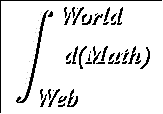Derivatives of Inverse Functions

Suggested Prerequesites:
Inverse Functions, Implicit Differentiation, Chain Rule

Sometimes it may be more convenient or even necessary to find the derivativebased on the knowledge or condition that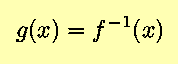for some function f(t), or, in other words, that g(x) is the inverse of f(t) = x. Then, recognizing that t and g(x) represent the same quantity, and remembering the Chain Rule,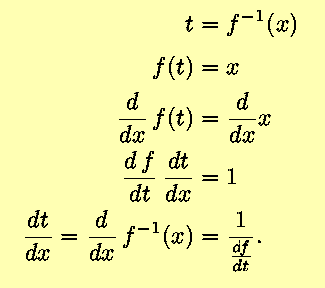Using Leibniz's fraction notation for derivatives, this result becomes somewhat obvious;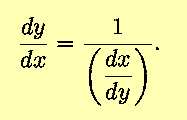As with the chain rule, the Leibnitz notation can often provide insight that can be confirmed using the definition of the derivative or previously proven results.

What the above does not indicate, and which will be shown via the following examples, is that when the derivative of an inverse function is desired as a function of (in the above and following cases) the independent variable x, the derivative dx/dy must be solved for x

### Some examples:

The following examples will be confined to those functions that can be differentiated by use of the Power Rule and the Chain Rule in combination.
• First, consider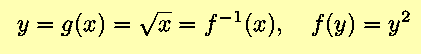We know from the Power Rule, with n=2, that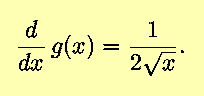Equivalently,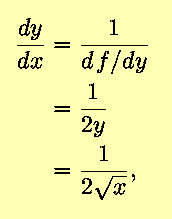as before.
• The above may be generalized; for nonzero n,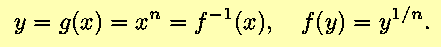Then,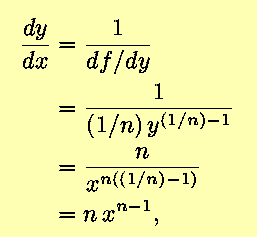in agreement with the Power Rule.

It may seem that the formalism doesn't add much to our toolkit for differentiation, and indeed, the above examples do not make a great case for memorizing the rule for differentation of inverse functions. However, when more complicated functions are considered, the use of the chain rule and implicit differentiation allows derivatives to be calculated with relative ease. Examples will be given in the appropriate sections of this hypertext.
Back to the Calculus page | Back to the World Web Math Categories Page

watko@mit.edu